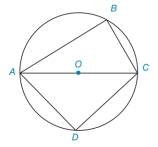Chapter 1.4, Problem 41E### Elementary Geometry for College St...

6th Edition
Daniel C. Alexander + 1 other
ISBN: 9781285195698

#### Solutions

Chapter
Section### Elementary Geometry for College St...

6th Edition
Daniel C. Alexander + 1 other
ISBN: 9781285195698
Textbook Problem
1 views

# Refer to the circle with centre O .a) Use a protractor to find m ∠ B .b) Use a protractor to find m ∠ D .c) Compare results in parts (a) and (b).To determine

a)

To find:

The measure of B.

Explanation

Given:

The given figure is a circle with centre O,

Approach:

The given figure is a circle with centre O,

Figure (1)

To determine

b)

To find:

The measure of D.

To determine

c)

To compare:

The measure of B and D.

### Still sussing out bartleby?

Check out a sample textbook solution.

See a sample solution

#### The Solution to Your Study Problems

Bartleby provides explanations to thousands of textbook problems written by our experts, many with advanced degrees!

Get Started

#### Evaluate the integrals in Problems 7-36. Check your results by differentiation. 13.

Mathematical Applications for the Management, Life, and Social Sciences

#### Find the inverse function of f(x)x2+2forx0.

Calculus: An Applied Approach (MindTap Course List)

#### The area of the region bounded by , , and r = sec θ is:

Study Guide for Stewart's Single Variable Calculus: Early Transcendentals, 8th